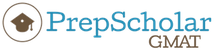## OG What is the area of triangular region ABC above?

##### This topic has expert replies
Master | Next Rank: 500 Posts
Posts: 394
Joined: 02 Jul 2017
Thanked: 1 times
Followed by:4 members

### OG What is the area of triangular region ABC above?

by AbeNeedsAnswers » Thu Aug 24, 2017 8:36 pm

00:00

A

B

C

D

E

## Global StatsWhat is the area of triangular region ABC above?

(1) The product of BD and AC is 20.
(2) x = 45

A

### GMAT/MBA Expert

Legendary Member
Posts: 503
Joined: 20 Jul 2017
Thanked: 86 times
Followed by:14 members
GMAT Score:770
by ErikaPrepScholar » Wed Aug 30, 2017 8:44 am

00:00

A

B

C

D

E

## Global Stats

This question asks us to determine whether or not we can solve for the area of triangle ABC. We should recognize that we will probably be dealing with the equation for area of a triangle: A = 1/2(bh). If we can solve this equation, we can solve the problem.

Statement 1
We know that BD is the height of our triangle and that AC is the base of our triangle. So the product of BD and AC = bh = 20. This means that we could plug 20 in for bh in our area equation to solve for A. Statement 1 is sufficient. We can eliminate answers B, C, and E.

Statement 2
If x = 45, we know that ABD is a 45-45-90 triangle, which means that angle ABD is also 45 degrees and that AD = BD. However, we still don't know a bunch of things.
1. We don't know how long AD and BD are-they could both be 1 unit long, they could both be 100 units long, they could both be 1000 units long. BD is our height and AD is part of our base, so changing these numbers has a pretty big impact on area.
2. We don't know anything about the length of CD-the other half of our base. It's easy to assume based on the diagram that angle BCD is 45 degrees, and thus CD is the same length as AD ... but we don't actually know that. CD could be way longer or way shorter than AD. Again, changing this length changes our base and thus changes area.
Statement 2 is NOT sufficient. A is correct.Erika John - Content Manager/Lead Instructor
https://gmat.prepscholar.com/gmat/s/

Get tutoring from me or another PrepScholar GMAT expert: https://gmat.prepscholar.com/gmat/s/tutoring/

Learn about our exclusive savings for BTG members (up to 25% off) and our 5 day free trial

Check out our PrepScholar GMAT YouTube channel, and read our expert guides on the PrepScholar GMAT blog

### GMAT/MBA Expert

Elite Legendary Member
Posts: 10392
Joined: 23 Jun 2013
Location: Palo Alto, CA
Thanked: 2867 times
Followed by:508 members
GMAT Score:800
by [email protected] » Wed Aug 30, 2017 10:01 am

00:00

A

B

C

D

E

## Global Stats

Hi AbeNeedsAnswers,

We're asked to figure out the area of triangular region ABC above. For that, we'll need the Area formula:

Area = (1/2)(Base)(Height)

So to answer this question, we either need the base and height of triangle ABC or we need the areas of the two smaller triangles.

(1) The product of BD and AC is 20.

Fact 1 tells us that the product of the height (BD) and the base (AC) = 20, so all we have to do is multiply that by 1/2 to get the area.... (1/2)(20) = 10
Fact 1 is SUFFICIENT

2) X = 45

This Fact tells us NOTHING about any of the side lengths, so there's no way to determine the area.
Fact 2 is INSUFFICIENT

Final Answer: A

GMAT assassins aren't born, they're made,
Rich

### GMAT/MBA Expert

GMAT Instructor
Posts: 6299
Joined: 25 Apr 2015
Location: Los Angeles, CA
Thanked: 43 times
Followed by:25 members

### Re: OG What is the area of triangular region ABC above?

by [email protected] » Fri May 14, 2021 6:28 am

00:00

A

B

C

D

E

## Global Stats

AbeNeedsAnswers wrote:
Thu Aug 24, 2017 8:36 pmWhat is the area of triangular region ABC above?

(1) The product of BD and AC is 20.
(2) x = 45

A
Solution:

Question Stem Analysis:

We need to determine the area of triangle ABC. Notice that AC is the base and the BD is the height of the triangle, therefore the area of triangle ABC is ½ x AC x BD.

Statement One Alone:

Since we are given that AC x BD = 20, the area of triangle ABC is ½ x 20 = 10. Statement one alone is sufficient.

Statement Two Alone:

Knowing angle A is 45 degrees does not allow us to determine the area of triangle ABC. Statement two is not sufficient.

Answer: A

• Page 1 of 1